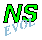## Bessel monopole on a beta-plane

Details on the computation

```Initialisation
Monopole:    Bessel monopole of radius 0.5 and strength 4
centred at (+3,-3)
Tracers:     single tracer point at the centre of the vortex
Computation: domain: X = (-10, ..., 10), Y = (-10, ..., 10)
no. of grid points from wall to wall: (257, 257)
boundary conditions: stress-free along all walls
standard Arakawa scheme used
rotation effects: f0 = 0, beta = 0.3, gamma = 0
no bottom topography
solver for streamfunction: FACR
viscosity: nu = 10^-3 (i.e. Re=1000 at T=0)
time step: dt = 0.05
final time: T = 50
```